# Subtraction With the Stamp Game

Subtraction With the Stamp Game
Math - Arithmetical Operations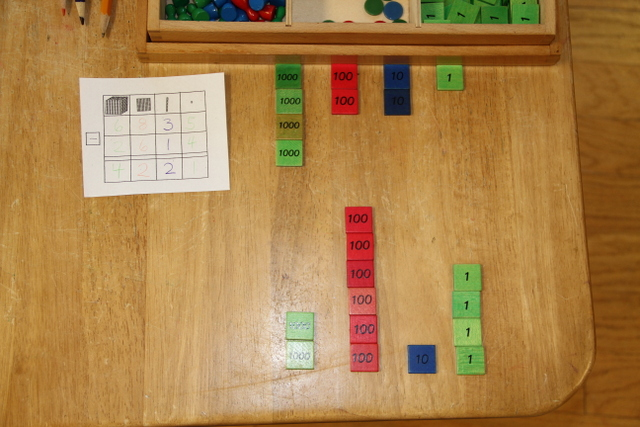LevelPrimary
Age5+
PrerequisitesUnderstanding of Decimal System
MaterialsStamp Game Box
pencil
green pencil
blue pencil
red pencil

This activity teaches the child to do subtraction problems at a higher level of abstraction.

## Presentations

### Static Subtraction

1. Take the material to a table. No divider line is needed for subtraction.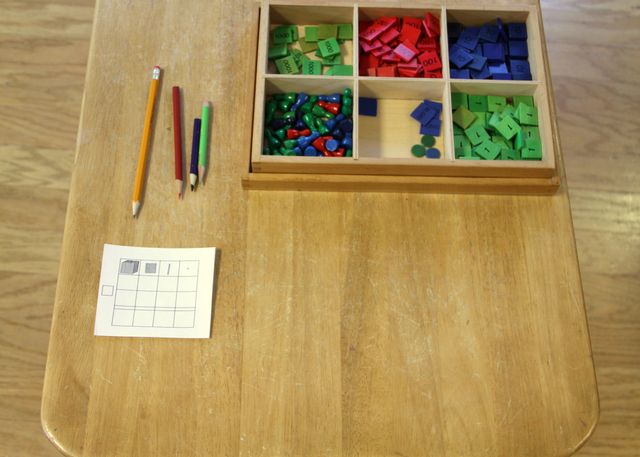2. Write out the problem.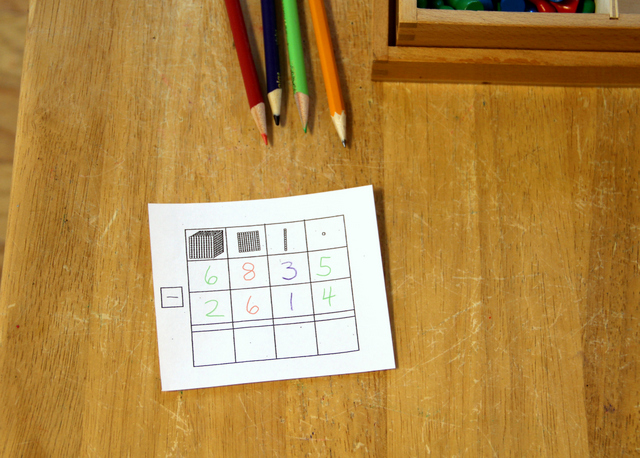3. Lay out the stamps for the first number (the minuend).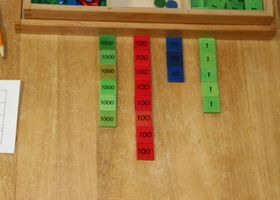4. Slide down the unit stamps for the second number (the subtrahend). Record the amount of unit stamps still left at the top.
Then do the same for the tens, then the hundreds, then the thousands.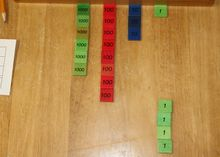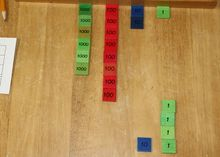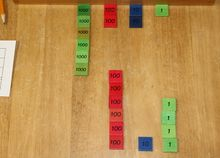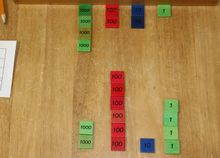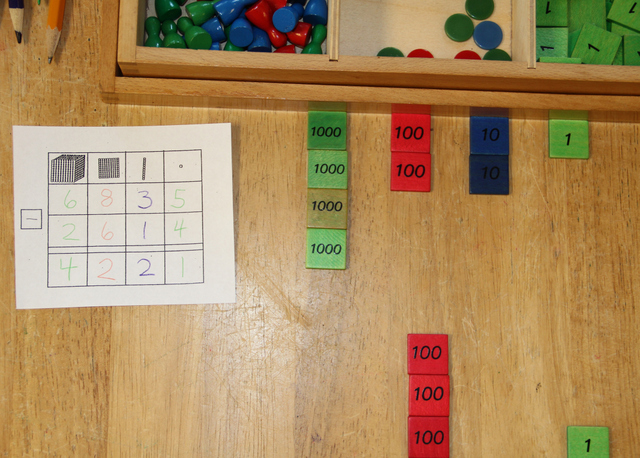### Dynamic Subtraction

1. step one
2. step two, etc.

## Material

Prices and links last checked: 14 May 2020

Absorbent Montessori \$47.00
Affordable Montessori \$33.00
Alison's \$36.75
Bruins \$89.00
E & O \$48.00
IFIT \$28.00